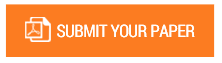Influence of Learning Model and Early Ability to Ability of Mathematics Problems on Class V Sd(Experimental Study on Grade V Students at SDN Kecamatan

Authors : Afidatus Sadiyah, Anton Noornia, Totok Bintoro.

Volume/Issue : Volume 3 - 2018, Issue 8 - August

Scribd : https://goo.gl/x8vnTP

Thomson Reuters ResearcherID : https://goo.gl/3bkzwv

This study aims to determine the effect of the learning model and early ability to solve the problem of mathematical problem. The research was conducted at SDN Kecamatan Duren Sawit, using experimental research design treatment by level 2 x 2. The result of research indicate that (1) There is difference of problem solving ability of mathematics between student which given model of cooperative learning type jigsaw with student which is given problem based learning model learning, (2) There is interaction effect between jigsaw type cooperative learning model and initial ability to problem-solving ability, (3) ability of problem solving of student with high initial ability with taught model of jigsaw type cooperative learning is higher than math problem-solving ability with taught learning problem-based learning model, (4) Ability to solve mathematical problems of students who have low initial ability with taught model jigsaw type cooperative learning is lower than the ability problem-solving mathematics with taught learning model problem-based learning.

Keywords : Problem based learning, jigsaw, initial ability, solution to problem.

#### CALL FOR PAPERS

Paper Submission Last Date
31 - May - 2022